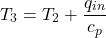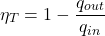# Diesel Cycle – Principles, Calculations, and Applications

#### Engineering

The diesel cycle is a thermodynamic cycle which undergoes internal combustion. It is similar to the Otto cycle. However, the main difference is that the fuel heats by the compression stroke rather than a spark plug. Likewise, the diesel cycle holds higher compression ratios than the Otto cycle. In this article, you will learn the principles, calculations, and applications of the diesel cycle.

## Principles of the Diesel Cycle

The cycle sees common use in of cars, trucks, and even marine engines. It holds one of the lowest fuel consumptions of all the cycles. The ability to produce a high-pressure ratio and high efficiency makes these engines commercially appealing. This cycle’s focus is to encourage engine knocking is what helps it hold higher pressure ratios than the Otto cycle.

The diesel cycle consists of four strokes, all of which play a different role in producing power from the fuel. Isentropic compression initiates the cycle as fuel and air combine in a cylinder. Next, an isobaric heat addition process occurs as the fuel ignites. The third step is an isentropic expansion that produces mechanical work. Finally, the process undergoes an isochoric heat rejection.

## Calculations of the Diesel Cycle

The diesel cycle operates differently from other kinds of internal combustion engines. Thus, analyzing the cycle’s efficiency looks somewhat different than other cycles. Prior to analysis, a pressure ratio, initial pressure, and initial temperature require definition. Throughout the cycle, instrumentation should monitor the temperature, pressure, and specific volume.

### Stage 1 ➔ 2 (Compression Stroke)

The second stage temp(T2) can be found by using the pressure ratio(r), the initial temperature(T1), and the air constant(k).Similarly, determination of the pressure(P2) at state 2 occurs from relating the initial pressure(P1), the pressure ratio(r), and the air value(k).### Stage 2 ➔ 3 (Heat Addition)

Depending on whether the third heat value(T3) or the heat supplied(qin) is defined, determines the equation to use. For either equation, users must know the specific heat at constant pressure(Cp).

With the third heat known, the equation to find supplied heat becomes:Alternatively, with heat supplied known and an unknown third temperature, the equation flips.Next, the compression ratio(rc) requires determination. This occurs by using the second temperature(T2) and the third temperature(T3).### Stage 3 ➔ 4 (Expansion Stroke)

Next, the calculation of the final temperature(T4) of the diesel cycle occurs to find efficiencies. Using the pressure ratio(r), the compression ratio(rc), the air value(k), and the third temperature(T3).### Stage 4 ➔ 1 (Heat Rejection)

Finally with all the intermediate values found the cycle as a whole can be analyzed. First, determine he heat rejected(qout) from the cycle. This occurs by using the specific heat at constant volume(Cv), the first temperature(T1), and the final temperature(T4).Next, determine the thermal efficiency of the overall cycle from the heat in(qin) and the heat out(qout).Now, determine the net work(Wnet) from using the heat in(qin) and the heat out(qout).The specific volume(v1) also needs to be found. Using the gas constant(R), the initial temperature(T1), and initial pressure(P1).Finally, find the mean effective pressure(MEP), a value that represents an engine’s capacity to do work. Find this number from the net work (Wnet), specific volume(v1) at state one, and the compression ratio(r).